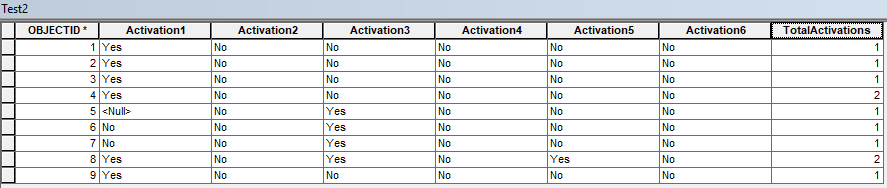# IF Statements based on several fields for Attribute Assistant

500
1
04-25-2019 06:33 AMOccasional Contributor II

Hello,

I am new to using attribute assistant and need some help. I need to be able to populate a field on the fly called Total Activations (field name TotalActivations) based on Activation1, Activation2, Activation3, Activation4, Activation5, and Activation6.

I can create a script in Python to do this, but not sure how to do it in Attribute Assistant. The reason why i would like to use Attribute Assistant is so that an user does not have to run a script tool every time an update is made. Here is what my table looks like:If the value is 'Yes' in any of the activation numbered fields, that takes the value of 1, any 'No' or <Null> values takes a value of 0. So the first line item would total 1.

My python script is the following:

``````import arcpy

poles = 'Test2'
fieldList = ("Activation1", "Activation2", "Activation3", "Activation4", "Activation5", "Activation6", "TotalActivations")

with arcpy.da.UpdateCursor(poles, fieldList) as cursor:
for row in cursor:
n = 0
if row == 'Yes':
a = 1
elif row == 'No' or row is None:
a = 0
if row == 'Yes':
b = 1
elif row == 'No' or row is None:
b = 0
if row == 'Yes':
c = 1
elif row == 'No' or row is None:
c = 0
if row == 'Yes':
d = 1
elif row == 'No' or row is None:
d = 0
if row == 'Yes':
e = 1
elif row == 'No' or row is None:
e = 0
if row == 'Yes':
f = 1
elif row == 'No' or row is None:
f = 0
n = a + b + c + d + e + f
row = n
cursor.updateRow(row)

‍‍‍‍‍‍‍‍‍‍‍‍‍‍‍‍‍‍‍‍‍‍‍‍‍‍‍‍‍‍‍‍‍‍‍‍‍``````

How do I translate this into Attribute Assistant?